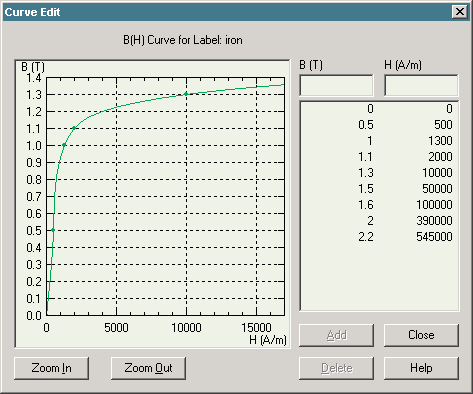QuickField

A new approach to field modelling
 Language: Global English Deutch Espanol Francais Italiano Danmark Ceske Chinese Pycckuü

>> >>

# Solenoid actuator parametric analysis

This is an example of the solenoid actuator simulation, performed with QuickField software.

Problem Type:
Axisymmetric problem of DC magnetics.

Geometry:Given:
Relative permeability of air and coil μ = 1;
Current density in the coil j = 4 A/mm2;
Core and plunger are made of non-linear ferromagnetic material:Problems:

1. Calculate the force vs. position characteristic
The plunger position can vary from 0 to 10 cm. With LabelMover we perform automatic geometry modifications with step of 1 cm. For each step the mechanical force is calculated.2. Study the current and plunger position influence on mechanical force
Nominal position of the plunger is 10+0.5 cm. Nominal current is 4 A/mm2+10%. With LabelMover Tolerance Analysis we perform variations and calculate the force automatically.3. Maximize the force
We can maximize the force by increasing the core window size. Thus more coil turns can be added to provide greater force. At the same time the core reluctance increases. Let's find the core window size that provides maximal force.
This can be easily done with LabelMover Optimization. The optimized core window height should be 71.75 mm (initially it was 40 mm).Video:
Serial calculations with LabelMover
Tolerance analysis with LabelMover
Optimization with LabelMover

• View simulation report in PDF
• Download simulation files (files may be viewed using any QuickField Edition).